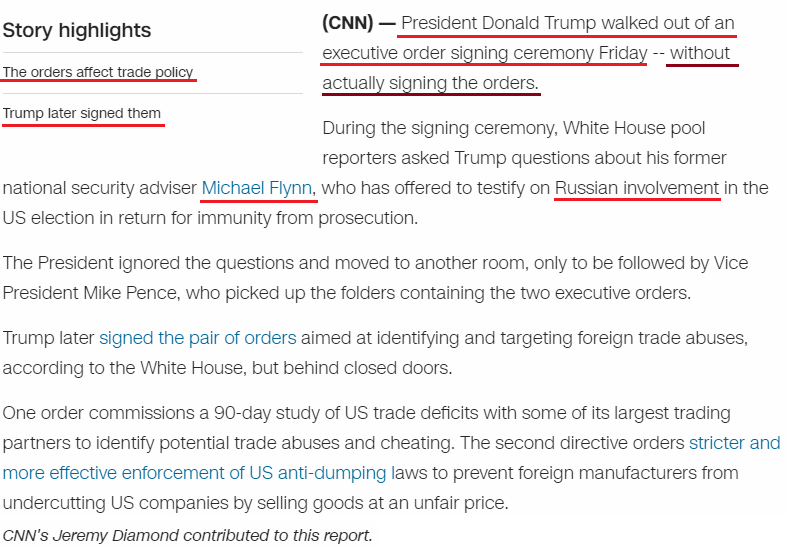CipherApr 2, 2017

CNN - Trump refuses to sign executive orders - Michael Flynn connection

http://edition.cnn.com/2017/03/31/politics/donald-trump-executive-order-signing-walk-out/index.html

Trump and Flynn have very strong connection to this date which is probably the reason for this story...They have a 49 second video connecting to the title (49th prime number is 227)Note how the first thing they write is split in 2 parts..The title gives us Pi (22/7 = 3.14) and the first thing they write sums to 360 connecting to 360° of a circle
three hundred sixty = 20+8+18+5+5 + 8+21+14+4+18+5+4 + 19+9+24+20+25 = 227 (Ordinal)This story is coming on 4/2/2017 - 4+2+20+17 = 43 - Forty two = 6+6+9+2+7 + 2+5+6 = 43 (Reduction)
Russia = 9+3+10+10+9+1 = 42 (S Exception)
Here is how Michael Flynn ties into thisHe's the 25th national security advisor - Trump = 2+9+3+4+7 = 25 (Reduced), Mike = 5+9+7+4 = 25 (Reverse Reduced) Pence = 7+5+5+3+5 = 25 (Reduced)
He was fired on 2/13/2017 - 2+13+2+0+1+7 = 25
On 13th of February Romans celebrated a holiday known as "Lupercalia" = 3+3+7+5+9+3+1+3+9+1 = 44 (Reduced)", 2/13 is the 44th day of the year  (Trump is the 44th person to be the president), Trump refused to sign executive orders on March 31st, a date with 44 numerology (3/31/2017 - 3+31+2+0+1+7 = 44)
"executive orders" = 5+24+5+3+21+20+9+22+5 + 15+18+4+5+18+19 = 193 (Ordinal)I can't find the day of Flynn's birth but we know that he's born in December = 5+4+6+4+5+7+4+9 = 44 (Reverse Reduced)

From the day when Trump got into office (1/20/2017) to the release of this story is 2 months and 13 days, connecting to 2/13 and Michael FlynnTrump's birthday is coming on the 74th day from the release of this story which is 2 months and 13 days